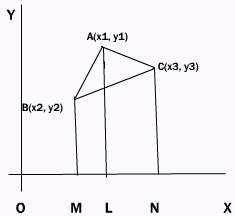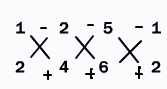Email us to get an instant 20% discount on highly effective K-12 Math & English kwizNET Programs!

#### Online Quiz (WorksheetABCD)

Questions Per Quiz = 2 4 6 8 10

### High School Mathematics - 29.18 Area of a triangle

 To find the area of triangle whose vertices are given.Let A(x1, y1), B(x2, y2) and C(x3, y3) be the vertices of the triangle. From A,B,C draw the perpendiculars AL, BM and CN on the x axis. Area of triangle ABC = area of trap ABML + trap ALNC - trap BMNC = 1/2[MB+LA]ML + 1/2[LA+CN]LN - 1/2[MB+NC]MN = 1/2(y2+y1) (x1-x2) + 1/2(y1+y3)(x3-x1) - 1/2(y2+y3)(x3-x2) = 1/2[x1(y2-y3)+x2(y3-y1)+x3(y1-y2)] Alternatively 1/2Example: Find the area of triangle whose vertices are (1,3), (2,4) and (5,6) Write out the x terms and y terms as shown. Multiply as shown. Divide the result by 2.1/2[1x4-2x3+2x6-5x4+5x3-1x6] = -1/2 Changing the sign, the area of the triangle in magnitude is 1/2. Directions: Solve the following.
 Q 1: The area of a triangle having vertices are (15,1), (x,3) and (2,7) is 18 sq.units. Find x.12510 Q 2: Find the area of the triangle whose vertices are (4,2), (4,5) and (-2,2).91112 Q 3: Find the area of the triangle the coordinates of the mid-points of whose side are (3,-1), (5,3) and (1,-3) respectively.24168 Q 4: Find the area of the triangle whose vertices are (2,7), (3,-1) and (-5,6).53/457/255/2 Q 5: Find the area of the triangle whose vertices are (0,0), (-2,3) and (10,7).252215 Q 6: Find the area of the triangle whose vertices are (-8,-2), (-4,-6) and (-1,5).232825 Q 7: Show that the lines 7x-2y+10=0, 7x+2y-10=0, y+2=0 form an isosceles triangle and find its area.121423 Q 8: If the centroid of triangle is (1,4) and two of the vertices are (4,-3) and (-9,7), find the area of a triangle.23183/2183 Question 9: This question is available to subscribers only! Question 10: This question is available to subscribers only!

#### Subscription to kwizNET Learning System offers the following benefits:

• Unrestricted access to grade appropriate lessons, quizzes, & printable worksheets
• Instant scoring of online quizzes
• Progress tracking and award certificates to keep your student motivated
• Unlimited practice with auto-generated 'WIZ MATH' quizzes